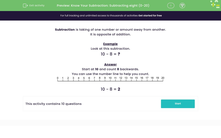Know Your Subtraction: Subtracting eight (0-20)

In this worksheet, students subtract eight from a number between 0 and 20.Key stage:  KS 1

Curriculum topic:   Number: Addition and Subtraction

Curriculum subtopic:   Add and Subtract to 20

Difficulty level:Worksheet Overview

Subtraction is taking of one number or amount away from another.

Example

Look at this subtraction.

10 - 8 = ?

Start at 10 and count 8 backwards.10 - 8 = 2

What is EdPlace?

We're your National Curriculum aligned online education content provider helping each child succeed in English, maths and science from year 1 to GCSE. With an EdPlace account you’ll be able to track and measure progress, helping each child achieve their best. We build confidence and attainment by personalising each child’s learning at a level that suits them.

Get started